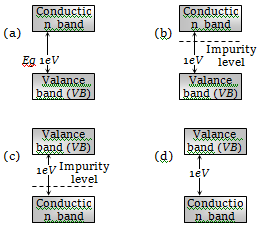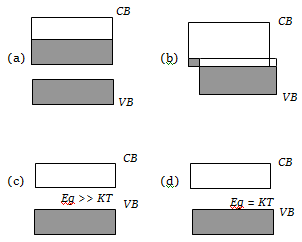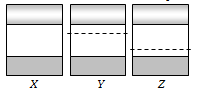Which of the following statements is true for an N-type semi-conductor

(a) The donor level lies closely below the bottom of the conduction band

(b) The donor level lies closely above the top of the valence band

(c) The donor level lies at the halfway mark of the forbidden energy gap

(d) None of above

Concept Questions :-

Types of semiconductors

Difficulty Level:

Choose the correct statement

(a) When we heat a semiconductor its resistance increases
(b) When we heat a semiconductor its resistance decreases
(c) When we cool a semiconductor to 0 K then it becomes super conductor
(d) Resistance of a semiconductor is independent of temperature

Concept Questions :-

Types of semiconductors
High Yielding Test Series + Question Bank - NEET 2020

Difficulty Level:

Intrinsic semiconductor is electrically neutral. Extrinsic semiconductor having large number of current carriers would be

(a) Positively charged

(b) Negatively charged

(c) Positively charged or negatively charged depending upon the type of impurity that has been added

(d) Electrically neutral

Concept Questions :-

Types of semiconductors
High Yielding Test Series + Question Bank - NEET 2020

Difficulty Level:

If ${\mathrm{n}}_{\mathrm{e}}$ and ${\mathrm{v}}_{\mathrm{d}}$ be the number of electrons and drift velocity in a semiconductor. When the temperature is increased

(a) ${\mathrm{n}}_{\mathrm{e}}$ increases and ${\mathrm{v}}_{\mathrm{d}}$ decreases
(b) ${\mathrm{n}}_{\mathrm{e}}$ decreases and ${\mathrm{v}}_{\mathrm{d}}$ increases
(c) Both ${\mathrm{n}}_{\mathrm{e}}$ and ${\mathrm{v}}_{\mathrm{d}}$ increases
(d) Both ${\mathrm{n}}_{\mathrm{e}}$ and ${\mathrm{v}}_{\mathrm{d}}$ decreases

Concept Questions :-

Types of semiconductors
High Yielding Test Series + Question Bank - NEET 2020

Difficulty Level:

The energy gap of silicon is 1.14 eV. The maximum wavelength at which silicon will begin absorbing energy is

(a) 10888 Å                    (b) 1088.8 Å
(c) 108.88 Å                   (d) 10.888 Å

Concept Questions :-

Energy band theory
High Yielding Test Series + Question Bank - NEET 2020

Difficulty Level:

Which of the following energy band diagram shows the N-type semiconductorConcept Questions :-

Energy band theory
High Yielding Test Series + Question Bank - NEET 2020

Difficulty Level:

The mobility of free electrons is greater than that of free holes because

(a) They carry negative charge

(b) They are light

(c) They mutually collide less

(d) They require low energy to continue their motion

Concept Questions :-

Types of semiconductors

Difficulty Level:

Which of the energy band diagrams shown in the figure corresponds to that of a semiconductorConcept Questions :-

Energy band theory
High Yielding Test Series + Question Bank - NEET 2020

Difficulty Level:

The energy band diagrams for three semiconductor samples of silicon are as shown. We can then assert that(a) Sample X is undoped while samples Y and Z have been doped with a third group and a fifth group impurity respectively
(b) Sample X is undoped while both samples Y and Z have been doped with a fifth group impurity
(c) Sample X has been doped with equal amounts of third and fifth group impurities while samples Y and Z are undoped
(d) Sample X is undoped while samples Y and Z have been doped with a fifth group and a third group impurity respectively

Concept Questions :-

Energy band theory
High Yielding Test Series + Question Bank - NEET 2020

Difficulty Level:

In a semiconducting material, the mobilities of electrons and holes are ${\mathrm{\mu }}_{\mathrm{e}}$ and ${\mathrm{\mu }}_{\mathrm{h}}$ respectively. Which of the following is true?
(a) ${\mathrm{\mu }}_{\mathrm{e}}$>${\mathrm{\mu }}_{\mathrm{h}}$               (b)  ${\mathrm{\mu }}_{\mathrm{e}}$<${\mathrm{\mu }}_{\mathrm{h}}$
(c)  ${\mathrm{\mu }}_{\mathrm{e}}$${\mathrm{\mu }}_{\mathrm{h}}$             (d)  ${\mathrm{\mu }}_{\mathrm{e}}$<0; ${\mathrm{\mu }}_{\mathrm{h}}$>0

Concept Questions :-

Types of semiconductors